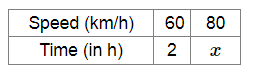# Tick (✓) the correct answer

Question:

A car takes 2 hours to reach a destination by travelling at 60 km/hr. How long will it take while travelling at 80 km/hr?

(a) 1 hr 30 min

(b) 1 hr 40 min

(c) 2 hrs 40 min

(d) none of these

Solution:

(a) 1 h 30 min

Let h be the time taken by the car travelling at 80 km/hr.The greater the speed, the lesser will be the time taken.

So, it is a case of inverse proportion.

Now, $60 \times 2=80 \times x$

$\Rightarrow x=\frac{120}{80}$

$\Rightarrow x=1.5$ Therefore, the car will take $1 \mathrm{~h} 30 \mathrm{~min}$ to reach its destination if it travels at a speed of $80 \mathrm{~km} / \mathrm{h} .$